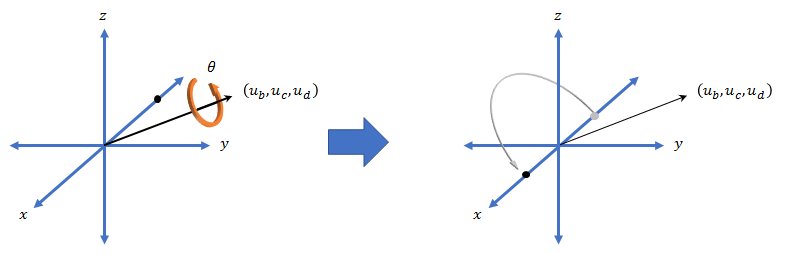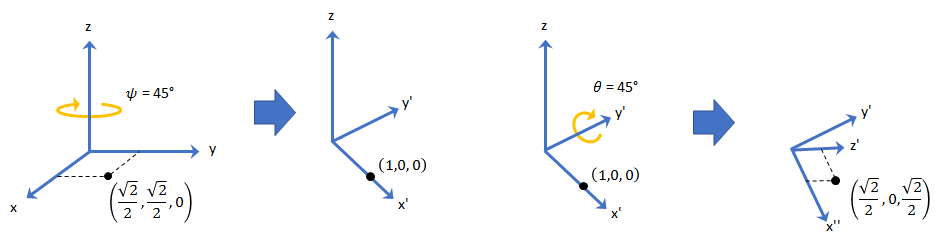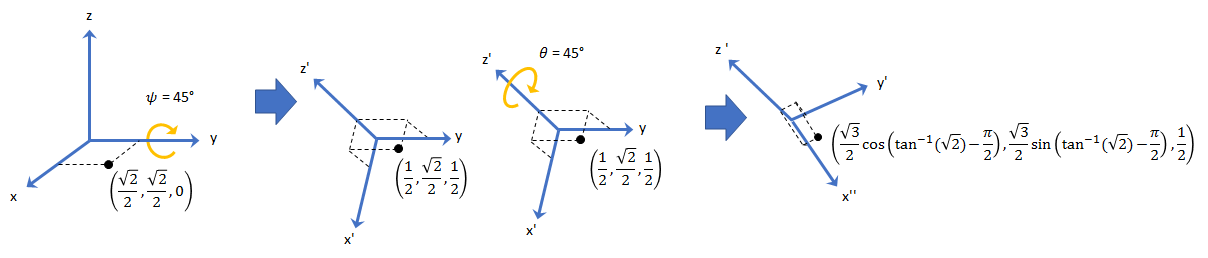# quaternion

Create a quaternion array

## Description

A quaternion is a four-part hyper-complex number used in three-dimensional rotations and orientations.

A quaternion number is represented in the form $a+b\text{i}+c\text{j}+d\text{k}$, where a, b, c, and d parts are real numbers, and i, j, and k are the basis elements, satisfying the equation: i2 = j2 = k2 = ijk = −1.

The set of quaternions, denoted by H, is defined within a four-dimensional vector space over the real numbers, R4. Every element of H has a unique representation based on a linear combination of the basis elements, i, j, and k.

All rotations in 3-D can be described by an axis of rotation and angle about that axis. An advantage of quaternions over rotation matrices is that the axis and angle of rotation is easy to interpret. For example, consider a point in R3. To rotate the point, you define an axis of rotation and an angle of rotation.The quaternion representation of the rotation may be expressed as $q=\mathrm{cos}\left(\theta }{2}\right)+\mathrm{sin}\left(\theta }{2}\right)\left({u}_{b}\text{i}+{u}_{c}\text{j}+{u}_{d}\text{k}\right)$, where θ is the angle of rotation and [ub, uc, and ud] is the axis of rotation.

## Creation

### Syntax

``quat = quaternion()``
``quat = quaternion(A,B,C,D)``
``quat = quaternion(matrix)``
``quat = quaternion(RV,'rotvec')``
``quat = quaternion(RV,'rotvecd')``
``quat = quaternion(RM,'rotmat',PF)``
``quat = quaternion(E,'euler',RS,PF)``
``quat = quaternion(E,'eulerd',RS,PF)``

### Description

example

````quat = quaternion()` creates an empty quaternion.```

example

````quat = quaternion(A,B,C,D)` creates a quaternion array where the four quaternion parts are taken from the arrays `A`, `B`, `C`, and `D`. All the inputs must have the same size and be of the same data type.```

example

````quat = quaternion(matrix)` creates an N-by-1 quaternion array from an N-by-4 matrix, where each column becomes one part of the quaternion.```

example

````quat = quaternion(RV,'rotvec')` creates an N-by-1 quaternion array from an N-by-3 matrix of rotation vectors, `RV`. Each row of `RV` represents a rotation vector in radians.```

example

````quat = quaternion(RV,'rotvecd')` creates an N-by-1 quaternion array from an N-by-3 matrix of rotation vectors, `RV`. Each row of `RV` represents a rotation vector in degrees.```

example

````quat = quaternion(RM,'rotmat',PF)` creates an N-by-1 quaternion array from the 3-by-3-by-N array of rotation matrices, `RM`. `PF` can be either `'point'` if the Euler angles represent point rotations or `'frame'` for frame rotations.```

example

````quat = quaternion(E,'euler',RS,PF)` creates an N-by-1 quaternion array from the N-by-3 matrix, `E`. Each row of `E` represents a set of Euler angles in radians. The angles in `E` are rotations about the axes in sequence `RS`.```

example

````quat = quaternion(E,'eulerd',RS,PF)` creates an N-by-1 quaternion array from the N-by-3 matrix, `E`. Each row of `E` represents a set of Euler angles in degrees. The angles in `E` are rotations about the axes in sequence `RS`.```

### Input Arguments

expand all

Parts of a quaternion, specified as four comma-separated scalars, matrices, or multi-dimensional arrays of the same size.

Example: `quat = quaternion(1,2,3,4)` creates a quaternion of the form 1 + 2i + 3j + 4k.

Example: `quat = quaternion([1,5],[2,6],[3,7],[4,8])` creates a 1-by-2 quaternion array where ```quat(1,1) = 1 + 2i + 3j + 4k``` and ```quat(1,2) = 5 + 6i + 7j + 8k```

Data Types: `single` | `double`

Matrix of quaternion parts, specified as an N-by-4 matrix. Each row represents a separate quaternion. Each column represents a separate quaternion part.

Example: `quat = quaternion(rand(10,4))` creates a 10-by-1 quaternion array.

Data Types: `single` | `double`

Matrix of rotation vectors, specified as an N-by-3 matrix. Each row of `RV` represents the [X Y Z] elements of a rotation vector. A rotation vector is a unit vector representing the axis of rotation scaled by the angle of rotation in radians or degrees.

To use this syntax, specify the first argument as a matrix of rotation vectors and the second argument as the `'rotvec'` or `'rotvecd'`.

Example: `quat = quaternion(rand(10,3),'rotvec')` creates a 10-by-1 quaternion array.

Data Types: `single` | `double`

Array of rotation matrices, specified by a 3-by-3 matrix or 3-by-3-by-N array. Each page of the array represents a separate rotation matrix.

Example: ```quat = quaternion(rand(3),'rotmat','point')```

Example: ```quat = quaternion(rand(3),'rotmat','frame')```

Data Types: `single` | `double`

Type of rotation matrix, specified by `'point'` or `'frame'`.

Example: ```quat = quaternion(rand(3),'rotmat','point')```

Example: ```quat = quaternion(rand(3),'rotmat','frame')```

Data Types: `char` | `string`

Matrix of Euler angles, specified by an N-by-3 matrix. If using the `'euler'` syntax, specify `E` in radians. If using the `'eulerd'` syntax, specify `E` in degrees.

Example: ```quat = quaternion(E,'euler','YZY','point')```

Example: ```quat = quaternion(E,'euler','XYZ','frame')```

Data Types: `single` | `double`

Rotation sequence, specified as a three-element character vector:

• `'YZY'`

• `'YXY'`

• `'ZYZ'`

• `'ZXZ'`

• `'XYX'`

• `'XZX'`

• `'XYZ'`

• `'YZX'`

• `'ZXY'`

• `'XZY'`

• `'ZYX'`

• `'YXZ'`

Assume you want to determine the new coordinates of a point when its coordinate system is rotated using frame rotation. The point is defined in the original coordinate system as:

`point = [sqrt(2)/2,sqrt(2)/2,0];`
In this representation, the first column represents the x-axis, the second column represents the y-axis, and the third column represents the z-axis.

You want to rotate the point using the Euler angle representation [45,45,0]. Rotate the point using two different rotation sequences:

• If you create a quaternion rotator and specify the 'ZYX' sequence, the frame is first rotated 45° around the z-axis, then 45° around the new y-axis.

```quatRotator = quaternion([45,45,0],'eulerd','ZYX','frame'); newPointCoordinate = rotateframe(quatRotator,point)```
```newPointCoordinate = 0.7071 -0.0000 0.7071```• If you create a quaternion rotator and specify the 'YZX' sequence, the frame is first rotated 45° around the y-axis, then 45° around the new z-axis.

```quatRotator = quaternion([45,45,0],'eulerd','YZX','frame'); newPointCoordinate = rotateframe(quatRotator,point)```
```newPointCoordinate = 0.8536 0.1464 0.5000```Data Types: `char` | `string`

## Object Functions

 `angvel` Angular velocity from quaternion array `classUnderlying` Class of parts within quaternion `compact` Convert quaternion array to N-by-4 matrix `conj` Complex conjugate of quaternion `ctranspose, '` Complex conjugate transpose of quaternion array `dist` Angular distance in radians `euler` Convert quaternion to Euler angles (radians) `eulerd` Convert quaternion to Euler angles (degrees) `exp` Exponential of quaternion array `ldivide, .\` Element-wise quaternion left division `log` Natural logarithm of quaternion array `meanrot` Quaternion mean rotation `minus, -` Quaternion subtraction `mtimes, *` Quaternion multiplication `norm` Quaternion norm `normalize` Quaternion normalization `ones` Create quaternion array with real parts set to one and imaginary parts set to zero `parts` Extract quaternion parts `power, .^` Element-wise quaternion power `prod` Product of a quaternion array `randrot` Uniformly distributed random rotations `rdivide, ./` Element-wise quaternion right division `rotateframe` Quaternion frame rotation `rotatepoint` Quaternion point rotation `rotmat` Convert quaternion to rotation matrix `rotvec` Convert quaternion to rotation vector (radians) `rotvecd` Convert quaternion to rotation vector (degrees) `slerp` Spherical linear interpolation `times, .*` Element-wise quaternion multiplication `transpose, .'` Transpose a quaternion array `uminus, -` Quaternion unary minus `zeros` Create quaternion array with all parts set to zero

## Examples

collapse all

`quat = quaternion()`
```quat = 0x0 empty quaternion array ```

By default, the underlying class of the quaternion is a double.

`classUnderlying(quat)`
```ans = 'double' ```

You can create a quaternion array by specifying the four parts as comma-separated scalars, matrices, or multidimensional arrays of the same size.

Define quaternion parts as scalars.

```A = 1.1; B = 2.1; C = 3.1; D = 4.1; quatScalar = quaternion(A,B,C,D)```
```quatScalar = quaternion 1.1 + 2.1i + 3.1j + 4.1k ```

Define quaternion parts as column vectors.

```A = [1.1;1.2]; B = [2.1;2.2]; C = [3.1;3.2]; D = [4.1;4.2]; quatVector = quaternion(A,B,C,D)```
```quatVector=2×1 quaternion array 1.1 + 2.1i + 3.1j + 4.1k 1.2 + 2.2i + 3.2j + 4.2k ```

Define quaternion parts as matrices.

```A = [1.1,1.3; ... 1.2,1.4]; B = [2.1,2.3; ... 2.2,2.4]; C = [3.1,3.3; ... 3.2,3.4]; D = [4.1,4.3; ... 4.2,4.4]; quatMatrix = quaternion(A,B,C,D)```
```quatMatrix=2×2 quaternion array 1.1 + 2.1i + 3.1j + 4.1k 1.3 + 2.3i + 3.3j + 4.3k 1.2 + 2.2i + 3.2j + 4.2k 1.4 + 2.4i + 3.4j + 4.4k ```

Define quaternion parts as three dimensional arrays.

```A = randn(2,2,2); B = zeros(2,2,2); C = zeros(2,2,2); D = zeros(2,2,2); quatMultiDimArray = quaternion(A,B,C,D)```
```quatMultiDimArray = 2x2x2 quaternion array quatMultiDimArray(:,:,1) = 0.53767 + 0i + 0j + 0k -2.2588 + 0i + 0j + 0k 1.8339 + 0i + 0j + 0k 0.86217 + 0i + 0j + 0k quatMultiDimArray(:,:,2) = 0.31877 + 0i + 0j + 0k -0.43359 + 0i + 0j + 0k -1.3077 + 0i + 0j + 0k 0.34262 + 0i + 0j + 0k ```

You can create a scalar or column vector of quaternions by specify an N-by-4 matrix of quaternion parts, where columns correspond to the quaternion parts A, B, C, and D.

Create a column vector of random quaternions.

`quatParts = rand(3,4)`
```quatParts = 3×4 0.8147 0.9134 0.2785 0.9649 0.9058 0.6324 0.5469 0.1576 0.1270 0.0975 0.9575 0.9706 ```
`quat = quaternion(quatParts)`
```quat=3×1 quaternion array 0.81472 + 0.91338i + 0.2785j + 0.96489k 0.90579 + 0.63236i + 0.54688j + 0.15761k 0.12699 + 0.09754i + 0.95751j + 0.97059k ```

To retrieve the `quatParts` matrix from quaternion representation, use `compact`.

`retrievedquatParts = compact(quat)`
```retrievedquatParts = 3×4 0.8147 0.9134 0.2785 0.9649 0.9058 0.6324 0.5469 0.1576 0.1270 0.0975 0.9575 0.9706 ```

You can create an N-by-1 quaternion array by specifying an N-by-3 matrix of rotation vectors in radians or degrees. Rotation vectors are compact spatial representations that have a one-to-one relationship with normalized quaternions.

Create a scalar quaternion using a rotation vector and verify the resulting quaternion is normalized.

```rotationVector = [0.3491,0.6283,0.3491]; quat = quaternion(rotationVector,'rotvec')```
```quat = quaternion 0.92124 + 0.16994i + 0.30586j + 0.16994k ```
`norm(quat)`
```ans = 1.0000 ```

You can convert from quaternions to rotation vectors in radians using the `rotvec` function. Recover the `rotationVector` from the quaternion, `quat`.

`rotvec(quat)`
```ans = 1×3 0.3491 0.6283 0.3491 ```

Rotation Vectors in Degrees

Create a scalar quaternion using a rotation vector and verify the resulting quaternion is normalized.

```rotationVector = [20,36,20]; quat = quaternion(rotationVector,'rotvecd')```
```quat = quaternion 0.92125 + 0.16993i + 0.30587j + 0.16993k ```
`norm(quat)`
```ans = 1 ```

You can convert from quaternions to rotation vectors in degrees using the `rotvecd` function. Recover the `rotationVector` from the quaternion, `quat`.

`rotvecd(quat)`
```ans = 1×3 20.0000 36.0000 20.0000 ```

You can create an N-by-1 quaternion array by specifying a 3-by-3-by-N array of rotation matrices. Each page of the rotation matrix array corresponds to one element of the quaternion array.

Create a scalar quaternion using a 3-by-3 rotation matrix. Specify whether the rotation matrix should be interpreted as a frame or point rotation.

```rotationMatrix = [1 0 0; ... 0 sqrt(3)/2 0.5; ... 0 -0.5 sqrt(3)/2]; quat = quaternion(rotationMatrix,'rotmat','frame')```
```quat = quaternion 0.96593 + 0.25882i + 0j + 0k ```

You can convert from quaternions to rotation matrices using the `rotmat` function. Recover the `rotationMatrix` from the quaternion, `quat`.

`rotmat(quat,'frame')`
```ans = 3×3 1.0000 0 0 0 0.8660 0.5000 0 -0.5000 0.8660 ```

You can create an N-by-1 quaternion array by specifying an N-by-3 array of Euler angles in radians or degrees.

Use the `euler` syntax to create a scalar quaternion using a 1-by-3 vector of Euler angles in radians. Specify the rotation sequence of the Euler angles and whether the angles represent a frame or point rotation.

```E = [pi/2,0,pi/4]; quat = quaternion(E,'euler','ZYX','frame')```
```quat = quaternion 0.65328 + 0.2706i + 0.2706j + 0.65328k ```

You can convert from quaternions to Euler angles using the `euler` function. Recover the Euler angles, `E`, from the quaternion, `quat`.

`euler(quat,'ZYX','frame')`
```ans = 1×3 1.5708 0 0.7854 ```

Euler Angles in Degrees

Use the `eulerd` syntax to create a scalar quaternion using a 1-by-3 vector of Euler angles in degrees. Specify the rotation sequence of the Euler angles and whether the angles represent a frame or point rotation.

```E = [90,0,45]; quat = quaternion(E,'eulerd','ZYX','frame')```
```quat = quaternion 0.65328 + 0.2706i + 0.2706j + 0.65328k ```

You can convert from quaternions to Euler angles in degrees using the `eulerd` function. Recover the Euler angles, `E`, from the quaternion, `quat`.

`eulerd(quat,'ZYX','frame')`
```ans = 1×3 90.0000 0 45.0000 ```

Quaternions form a noncommutative associative algebra over the real numbers. This example illustrates the rules of quaternion algebra.

Quaternion addition and subtraction occur part-by-part, and are commutative:

`Q1 = quaternion(1,2,3,4)`
```Q1 = quaternion 1 + 2i + 3j + 4k ```
`Q2 = quaternion(9,8,7,6)`
```Q2 = quaternion 9 + 8i + 7j + 6k ```
`Q1plusQ2 = Q1 + Q2`
```Q1plusQ2 = quaternion 10 + 10i + 10j + 10k ```
`Q2plusQ1 = Q2 + Q1`
```Q2plusQ1 = quaternion 10 + 10i + 10j + 10k ```
`Q1minusQ2 = Q1 - Q2`
```Q1minusQ2 = quaternion -8 - 6i - 4j - 2k ```
`Q2minusQ1 = Q2 - Q1`
```Q2minusQ1 = quaternion 8 + 6i + 4j + 2k ```

You can also perform addition and subtraction of real numbers and quaternions. The first part of a quaternion is referred to as the real part, while the second, third, and fourth parts are referred to as the vector. Addition and subtraction with real numbers affect only the real part of the quaternion.

`Q1plusRealNumber = Q1 + 5`
```Q1plusRealNumber = quaternion 6 + 2i + 3j + 4k ```
`Q1minusRealNumber = Q1 - 5`
```Q1minusRealNumber = quaternion -4 + 2i + 3j + 4k ```

Multiplication

Quaternion multiplication is determined by the products of the basis elements and the distributive law. Recall that multiplication of the basis elements, i, j, and k, are not commutative, and therefore quaternion multiplication is not commutative.

`Q1timesQ2 = Q1 * Q2`
```Q1timesQ2 = quaternion -52 + 16i + 54j + 32k ```
`Q2timesQ1 = Q2 * Q1`
```Q2timesQ1 = quaternion -52 + 36i + 14j + 52k ```
`isequal(Q1timesQ2,Q2timesQ1)`
```ans = logical 0 ```

You can also multiply a quaternion by a real number. If you multiply a quaternion by a real number, each part of the quaternion is multiplied by the real number individually:

`Q1times5 = Q1*5`
```Q1times5 = quaternion 5 + 10i + 15j + 20k ```

Multiplying a quaternion by a real number is commutative.

`isequal(Q1*5,5*Q1)`
```ans = logical 1 ```

Conjugation

The complex conjugate of a quaternion is defined such that each element of the vector portion of the quaternion is negated.

`Q1`
```Q1 = quaternion 1 + 2i + 3j + 4k ```
`conj(Q1)`
```ans = quaternion 1 - 2i - 3j - 4k ```

Multiplication between a quaternion and its conjugate is commutative:

`isequal(Q1*conj(Q1),conj(Q1)*Q1)`
```ans = logical 1 ```

You can organize quaternions into vectors, matrices, and multidimensional arrays. Built-in MATLAB® functions have been enhanced to work with quaternions.

Concatenate

Quaternions are treated as individual objects during concatenation and follow MATLAB rules for array manipulation.

```Q1 = quaternion(1,2,3,4); Q2 = quaternion(9,8,7,6); qVector = [Q1,Q2]```
```qVector=1×2 quaternion array 1 + 2i + 3j + 4k 9 + 8i + 7j + 6k ```
```Q3 = quaternion(-1,-2,-3,-4); Q4 = quaternion(-9,-8,-7,-6); qMatrix = [qVector;Q3,Q4]```
```qMatrix=2×2 quaternion array 1 + 2i + 3j + 4k 9 + 8i + 7j + 6k -1 - 2i - 3j - 4k -9 - 8i - 7j - 6k ```
```qMultiDimensionalArray(:,:,1) = qMatrix; qMultiDimensionalArray(:,:,2) = qMatrix```
```qMultiDimensionalArray = 2x2x2 quaternion array qMultiDimensionalArray(:,:,1) = 1 + 2i + 3j + 4k 9 + 8i + 7j + 6k -1 - 2i - 3j - 4k -9 - 8i - 7j - 6k qMultiDimensionalArray(:,:,2) = 1 + 2i + 3j + 4k 9 + 8i + 7j + 6k -1 - 2i - 3j - 4k -9 - 8i - 7j - 6k ```

Indexing

To access or assign elements in a quaternion array, use indexing.

`qLoc2 = qMultiDimensionalArray(2)`
```qLoc2 = quaternion -1 - 2i - 3j - 4k ```

Replace the quaternion at index two with a quaternion one.

`qMultiDimensionalArray(2) = ones('quaternion')`
```qMultiDimensionalArray = 2x2x2 quaternion array qMultiDimensionalArray(:,:,1) = 1 + 2i + 3j + 4k 9 + 8i + 7j + 6k 1 + 0i + 0j + 0k -9 - 8i - 7j - 6k qMultiDimensionalArray(:,:,2) = 1 + 2i + 3j + 4k 9 + 8i + 7j + 6k -1 - 2i - 3j - 4k -9 - 8i - 7j - 6k ```

Reshape

To reshape quaternion arrays, use the `reshape` function.

`qMatReshaped = reshape(qMatrix,4,1)`
```qMatReshaped=4×1 quaternion array 1 + 2i + 3j + 4k -1 - 2i - 3j - 4k 9 + 8i + 7j + 6k -9 - 8i - 7j - 6k ```

Transpose

To transpose quaternion vectors and matrices, use the `transpose` function.

`qMatTransposed = transpose(qMatrix)`
```qMatTransposed=2×2 quaternion array 1 + 2i + 3j + 4k -1 - 2i - 3j - 4k 9 + 8i + 7j + 6k -9 - 8i - 7j - 6k ```

Permute

To permute quaternion vectors, matrices, and multidimensional arrays, use the `permute` function.

`qMultiDimensionalArray`
```qMultiDimensionalArray = 2x2x2 quaternion array qMultiDimensionalArray(:,:,1) = 1 + 2i + 3j + 4k 9 + 8i + 7j + 6k 1 + 0i + 0j + 0k -9 - 8i - 7j - 6k qMultiDimensionalArray(:,:,2) = 1 + 2i + 3j + 4k 9 + 8i + 7j + 6k -1 - 2i - 3j - 4k -9 - 8i - 7j - 6k ```
`qMatPermute = permute(qMultiDimensionalArray,[3,1,2])`
```qMatPermute = 2x2x2 quaternion array qMatPermute(:,:,1) = 1 + 2i + 3j + 4k 1 + 0i + 0j + 0k 1 + 2i + 3j + 4k -1 - 2i - 3j - 4k qMatPermute(:,:,2) = 9 + 8i + 7j + 6k -9 - 8i - 7j - 6k 9 + 8i + 7j + 6k -9 - 8i - 7j - 6k ```

## Extended Capabilities

### C/C++ Code GenerationGenerate C and C++ code using MATLAB® Coder™.